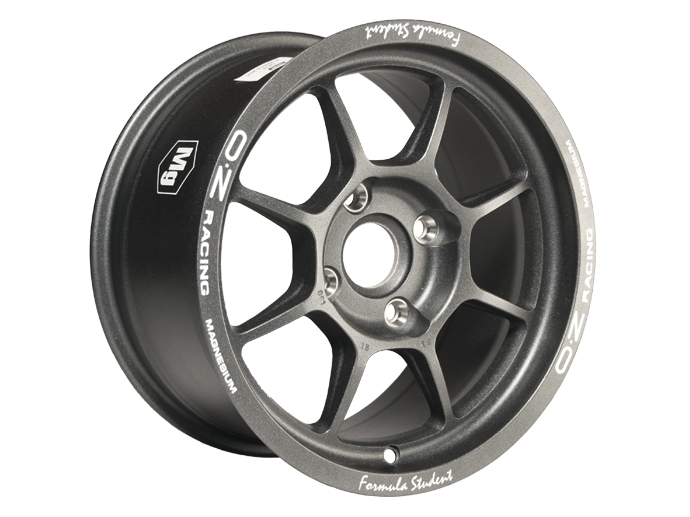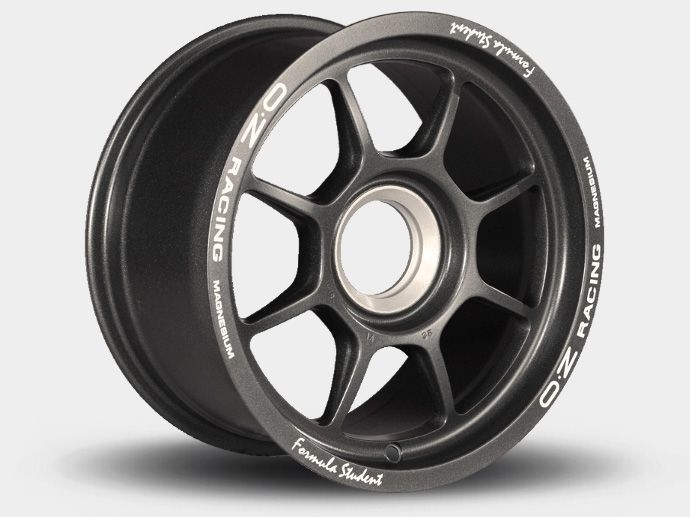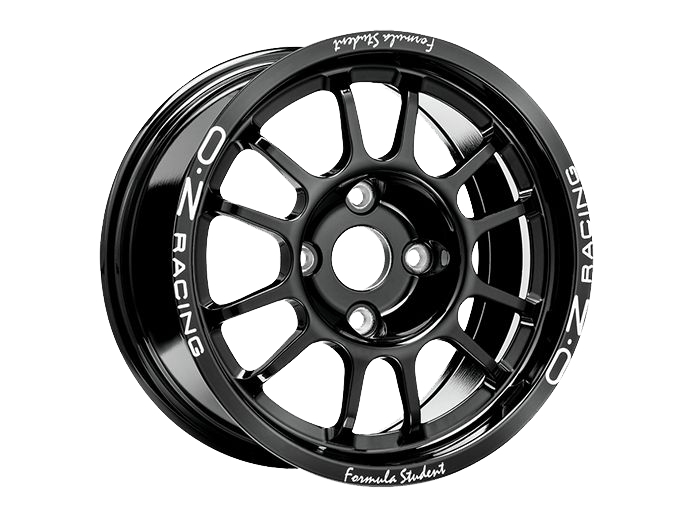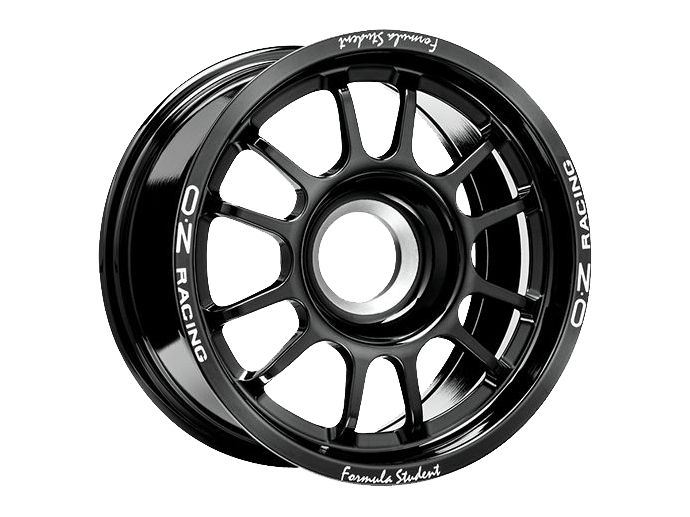Formula StudentOZ Formula Student Magnesium 4H wheelOZ Formula Student Magnesium CL wheelOZ Formula Student Magnesium CL 10" wheelOZ Formula Student Alluminium 4H wheel